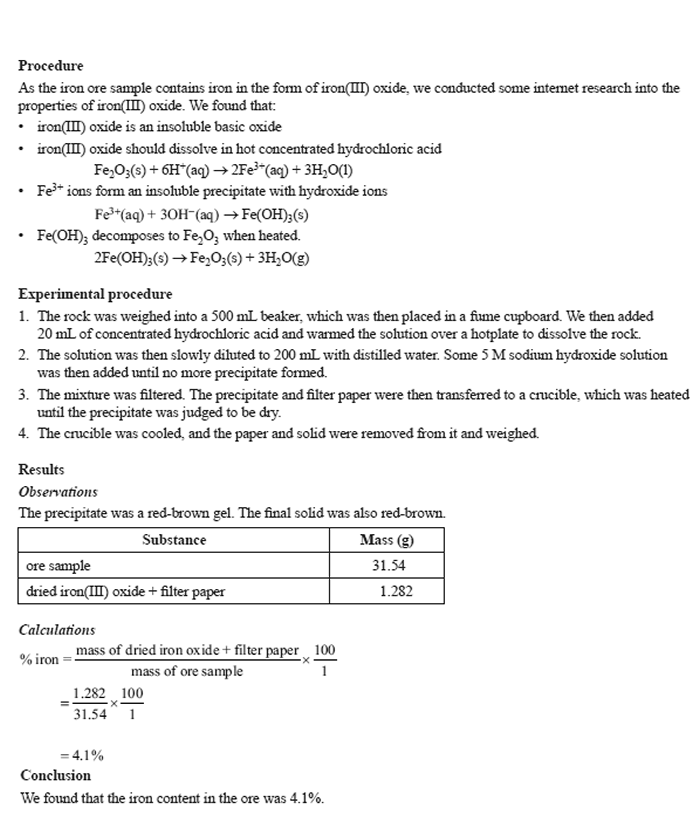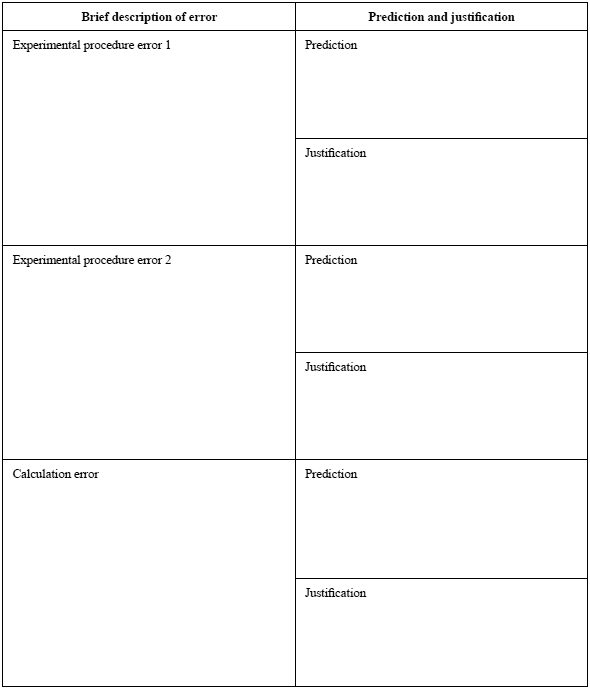Investigation (2015 VCE) Two Chemistry students were set the task of using gravimetric analysis to determine the percentage by mass of iron in an iron ore sample. They were informed that the small rock of iron ore they had been given as a sample only contained iron in the form of iron(III) oxide. Below is part of their report.The students' description of their experimental procedure and calculations contains some errors, which may include omissions. In the table provided below, briefly describe two errors in their experimental procedure and one error in their calculations. In each case, predict how the error would have affected their calculated value for the percentage of iron in the rock. Justify your answers. (Assume that the students recorded each step in their procedure and calculations.) Solution